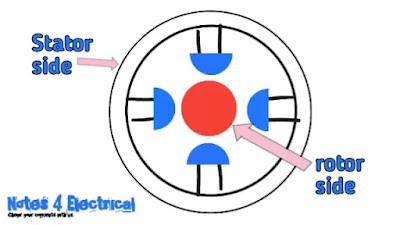# Speed control of induction motor,advantage and disadvantages of 3 phase induction motor

Hello guys, welcome to my blog. Hope you are all good and well.

• Advantages of three phase induction motors
• Speed control of induction motor.
As we have discussed about the various topics of induction motor in previous articles.

So let's understand first,

## Advantage of 3 phase induction motor: -

• Three phase induction motors are simple and rugged in construction.
• These are Cheaper in cost.
• These motors are Maintenance free.
• Induction motors Can be operated in a polluted environment.
• And the most important thing that these motors are self starting motor.
Now let’s come to understand,

## Speed control of induction motor: -

There are mainly two methods to control the speed of 3 phase induction motors are –

• Stator side method
• Rotor side method

So let’s discuss about stator side method.Fig.1 speed control of 3 phase induction motor

### 1. Stator side method: -

#### As the name suggest, something has done on the stator side to control the speed of three phase induction motors. So what’s done?

In stator side method the speed can be controlled by changing the applied voltage, frequency and number of poles.

• By changing the applied voltage: -

The speed can be changed by changing the applied voltage. As we increase the supply voltage the speed will decrease and if we decrease the supply voltage the speed will increase.

So we can say that the supply voltage is inversely proportional to the  speed.

Now a question arises here that how to control the voltage or change the supply voltage??

The supply voltage can be varied by two methods –
a) By using three phase auto transformer
b) By using a rheostat (variable resistance)

• By changing frequency: -

The synchronous speed is given by the formula,

Ns = 120f/P

Now, here we can clearly see that the synchronous speed of the induction motor is directly proportional to the frequency.

And therefore by changing the frequency we can change the speed.

If we increase the frequency, then the speed will also increase and vice versa.

Now how to control or change the frequency?

For this purpose, we generally used VFD or variable frequency device which is used to change the frequency.

Note: -
In actual, not only the frequency is changed, the supply voltage also varies with frequency.

So why speed is changed by changing the synchronous speed?

As guys we have discussed in the previous article that the rotating magnetic field of the stator is called synchronous speed.

And when we decrease the synchronous speed, i.e. speed of rotating magnetic field, then the rotating magnetic flux cuts less to the rotor conductors as compared to before.

So in this case it cuts less and produce less current in the rotor conductors as compared to before.

As the current will change, the torque will also change. And further, if torque will change, then the speed will also change.

And as here the synchronous speed decreases, therefore the speed of induction motor is also decreased. If we will increase the synchronous speed, the speed of induction motor will increase.

• By changing the number of poles: -
As we have seen that the synchronous speed is directly proportional to the frequency, whereas it is inversely proportional to the number of poles.

Therefore, by changing the number of poles we can change the speed of induction motor.

So a question ariseis here that how the poles are changed?
While motor poles had already constructed during the manufacturing of induction motor.

Is poles reconstruct again for changing the speed?

So guys during the manufacturing of induction motor the windings are placed on the stator poles and construct in such a way that it’s connection can be changed at any time by a switch.

This switch connects two consecutive windings to each other and make one pole.

Let us understand this by an example: -

Let assume there is an 3 phase induction motor which has four poles (having four poles on which separate windings are placed). Now when we want to change its no. of poles (to change its speed) then we will on the switch as this switch connects to consecutive windings to each other and make only one pole (winding) by these two windings.

So this four pole motor will convert into two pole motor. In other words, we can say that this switch combines two consecutive poles and made them only one pole.

Note: -
In induction motor, there will always be even number of poles, i.e. 2,4,6 etc.

Now let us discuss about the rotor side method.

### 2.Rotor side method: -

In this method, the rotor resistance is varied by connecting external resistance in the rotor with the help of slip rings.

Hence we know that with squirrel cage rotor type induction motor the rotor bars are short circuited by end rings permanently to each other and hence external resistance can not be connected in squirrel cage rotor type induction motor.

#### Therefore, this method is only suitable for speed control of slip ring induction rotor type induction motor.

So if we change the rotor resistance the current flowing though the rotor will also change and by changing this current the torque produced will also change which indirectly changes the speed of three phase induction motors.

So this was about speed control of  3 phase induction motor.

If you have any doubt then tell me in comment.

If you like the article then share with your friends

Thank you.

Hope article helps you.

Tags: -
Speed control of three phase induction motor pdf
Speed control of three phase slip ring induction motor